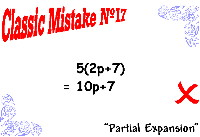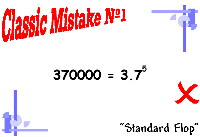Search IntMath
Close

# Classic math mistakes

By Murray Bourne, 21 Dec 2009

Here's a collection of mistakes in math. It's interesting these mistakes are common all over the world. It could be something to do with the crazy inconsistencies in the way math is written. (See my Towards more meaningful math notation.)

This is Mistake #17:And this is Mistake #01:You can download (free) PDFs of these in color and black and white. There's also an MP3 commentary on each one.

Don't miss the Freebies page, which has more posters.

### Comment Preview

HTML: You can use simple tags like <b>, <a href="...">, etc.

To enter math, you can can either:

1. Use simple calculator-like input in the following format (surround your math in backticks, or qq on tablet or phone):
a^2 = sqrt(b^2 + c^2)
(See more on ASCIIMath syntax); or
2. Use simple LaTeX in the following format. Surround your math with $$ and $$.
$$\int g dx = \sqrt{\frac{a}{b}}$$
(This is standard simple LaTeX.)

NOTE: You can mix both types of math entry in your comment.